Ch 2. Particle Force and Acceleration Multimedia Engineering Dynamics Rect.Coord. Normal/Tang. Coord. PolarCoord. Orbital Mechanics Computational Mechanics
 Chapter - Particle - 1. General Motion 2. Force & Accel. 3. Energy 4. Momentum - Rigid Body - 5. General Motion 6. Force & Accel. 7. Energy 8. Momentum 9. 3-D Motion 10. Vibrations Appendix Basic Math Units Basic Equations Sections Search eBooks Dynamics Fluids Math Mechanics Statics Thermodynamics Author(s): Kurt Gramoll ©Kurt GramollDYNAMICS - CASE STUDY SOLUTION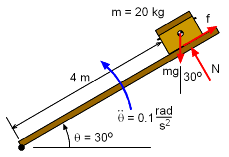Force Diagram Begin with a free-body diagram at 30°. Sum the forces in both the radial and transverse directions.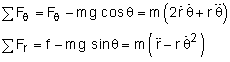In the transverse direction, θ, there is only one unknowns, Fθ (= N). All other terms can be determined from the basic parameters given, mainly,      r = 4 mFθ = N = 20 (9.81) cos30 + 20 [ 0 + (4)(0.1) ]      N = 177.9 N Now the second radial force equation can be used to find the minimum static coefficient of friction required to keep the chest from sliding when the drawbridge reaches an angle of 30°: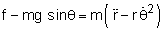where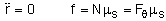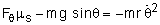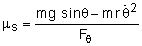The problem with this equation is dθ/dt is not known. To find it, the angular acceleration, a constant 0.1 rad/s2, needs to be integrated,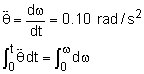ω(t) = 0.1 t = dθ/dt Now the problem is t is not known. By integrating it again, the angle, θ(t), can be determined,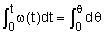θ(t) = 0.05 t2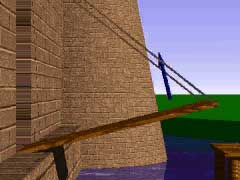Velocity Vector Animation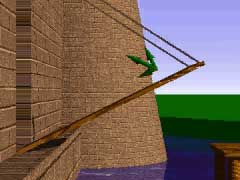Velocity Acceleration Animation The time required for the drawbridge to reach an angle of 30° is      θ(t) = 30o = 0.5237 rad = 0.1/2 t2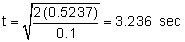Substitute t back into the ω(t) equation above to determine the angular velocity of the drawbridge when it reaches an angle of 30°:      ωt = 3.24 = 0.1 (3.236) = 0.3236 rad/s2 Now, that dθ/dt is known for 30°, the minimum static coefficient of friction is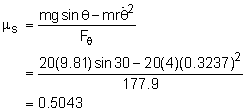Practice Homework and Test problems now available in the 'Eng Dynamics' mobile app
Includes over 400 problems with complete detailed solutions.
Available now at the Google Play Store and Apple App Store.